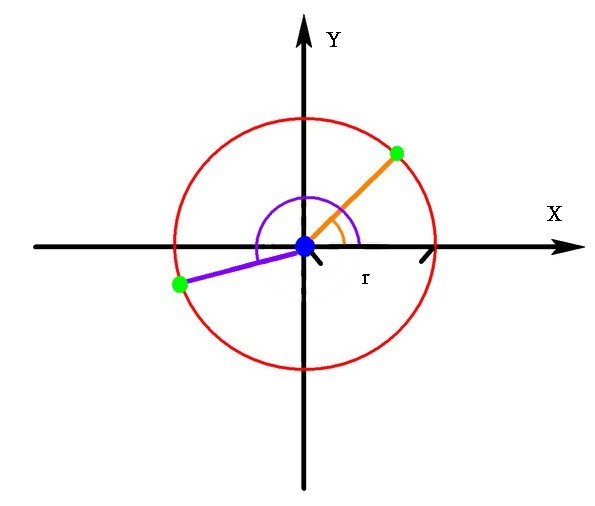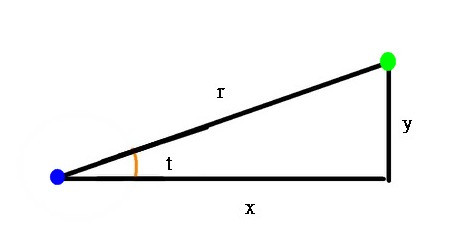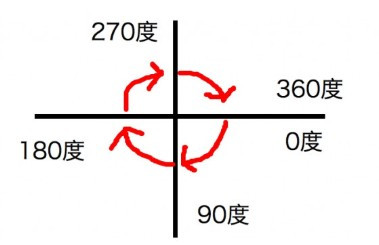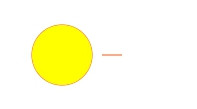#4

## [筆記][HTML][JavaScript]canvas的基本用法(4)-一點都不基本的極座標！

OK，讓我們搭配圖形，來簡單說說這是怎麼一回事：sinθ = y / r
cosθ = x / r

y = sinθ * r
x = cosθ * r

HTML

``````<canvas id="myCanvas"></canvas>
``````

JavaSript

``````let canvas = document.getElementById("myCanvas");
let ctx = canvas.getContext("2d");

//紀錄滑鼠位置
let mouse = {
x : 0,
y : 0,
};

//監聽滑鼠事件
mouse.x = event.pageX;
mouse.y = event.pageY;
});

//一個繪製的function
const draw = () =>{
//把視窗大小重新設定給canvas
canvas.height = window.innerHeight;
canvas.width = window.innerWidth;

ctx.clearRect(0, 0, canvas.width, canvas.height);

//之後繪製太陽
ctx.beginPath();
ctx.arc(mouse.x,mouse.y,30,0,Math.PI*2);
ctx.strokeStyle="#FF5511";
ctx.stroke();
ctx.fillStyle="#FFFF00";
ctx.fill();

//繪製光芒線條
lightLine();
};

//繪製光芒線條的function
const lightLine = () =>{

};
//每隔50毫秒重新繪製一次
setInterval('draw()',50);
``````1. `JavaScript`中的`Math.PI`是代表角度180度。
2. 在一個座標中，角度從0度，也就是貼著X軸開始，越下面角度越大，直到繞一圈回到0度，可以參照下圖：JavaScript

``````//繪製光芒線條的function
const lightLine = () =>{
//開始繪圖
ctx.beginPath();
//開始點為原點，滑鼠目前的所在座標
ctx.moveTo(mouse.x,mouse.y);

//從原點到某一極座標，用文章開頭說的公式帶入數字，設角度為0，r為60，取得X軸及Y軸
//求X的公式為x = cosθ * r
let X = Math.cos(0) * 60;
//求y的公式為x = sinθ * r
let Y = Math.sin(0) * 60;

//上方取得的X及Y為從原點到某一極點的距離，所以我們把原點的所在座標加上該數值
ctx.lineTo(mouse.x + X,mouse.y + Y);
ctx.stroke();
};
``````1. 半徑
2. 角度

JavaScript

``````const lightLine = () =>{
ctx.beginPath();
//把r設為40取x軸和y軸距離原點的距離
let X = Math.cos(0) * 40;
let Y = Math.sin(0) * 40;
//用原點加上該距離
ctx.moveTo(mouse.x + X,mouse.y + Y);

//結束點不變
X = Math.cos(0) * 60;
Y = Math.sin(0) * 60;
ctx.lineTo(mouse.x + X,mouse.y + Y);
ctx.stroke();
};
``````JavaScript

``````const lightLine = () =>{
ctx.beginPath()
//設定一個變數為角度
let t = 0;
//假設我們要畫夾角為17度的線段
t = 17
/*把計算cosθ和sinθ的切出來
並以角度t除上360度取得比例後在乘上Math.PI*2獲得角度
*/
let cosT = Math.cos((t/360) * (Math.PI*2))
let sinT = Math.sin((t/360) * (Math.PI*2))

let X = cosT * 40;
let Y = sinT * 40;
ctx.moveTo(mouse.x + X,mouse.y + Y);

X = cosT * 60;
Y = sinT * 60;
ctx.lineTo(mouse.x + X,mouse.y + Y);
ctx.stroke();
};
````````````const lightLine = () =>{
ctx.beginPath()

//把角度t放到迴圈中，從0開始到360度，每隔20度就畫繪製一條光線
for(let t=0;t<360;t+=20){
let cosT = Math.cos((t/360) * (Math.PI*2))
let sinT = Math.sin((t/360) * (Math.PI*2))
let X = cosT * 40;
let Y = sinT * 40;
ctx.moveTo(mouse.x + X,mouse.y + Y);
X = cosT * 60;
Y = sinT * 60;
ctx.lineTo(mouse.x + X,mouse.y + Y);
ctx.stroke();
};
};
``````